﻿ 矩阵乘法 – Hzao's Blog

# 矩阵乘法

#### 运算方法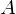矩阵和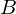矩阵乘积中的元素,等于矩阵第行的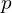个数和矩阵第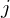列的个数分别相乘的和。 即：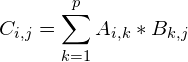#### 纯乘法的代码实现

1.计算函数。由于自己太弱了，加上时间有限，写了一份很水的代码。

#include<cstdio>
int A={
{1,2,3},
{4,5,6}
};
int B={
{1,2,3,4},
{5,6,7,8},
{9,10,11,12}
};
int c;
int n,m;
void mul(int a[],int b[],int n,int m,int p){
for(int i=0;i<n;++i){//n 乘积的行数
for(int j=0;j<m;++j){//m 乘积的列数
for(int k=0;k<p;++k){//p 即共有的维
c[i][j]+=a[i][k]*b[k][j];
}
printf("c[%d][%d]=%d\n",i,j,c[i][j]);
}
}
}
int main(){
mul(A,B,2,4,3);//计算A*B,并保存到c[]数组中
return 0;
}

2.有一种边读入边计算的写法：

	scanf("%lld%lld%lld",&n,&p,&m);
for(long long i=1;i<=n;++i){
for(long long j=1;j<=p;++j){
scanf("%lld",&a[i][j]);
}
}
for(long long i=1;i<=p;++i){
for(long long j=1;j<=m;++j){
scanf("%lld",&b[i][j]);
for(long long k=1;k<=n;++k){//此处k枚举的并非是公共维
ans[k][j]+=a[k][i]*b[i][j];
}
}
}

#### 用途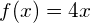之类的线性函数的推广。

——维基百科

1.其实蒟蒻的我并不知道怎么解线性方程组，但是对于矩阵加速线性变换有一些体会。让我们考虑一种比较特殊的矩阵乘法：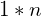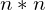。由定义可知，它们的乘积仍然是一个的矩阵。那么有什么用呢？我们可以借助快速幂的思想，利用矩阵乘法的分配律、结合律，降低时间复杂度。2.以后遇到再来补充。